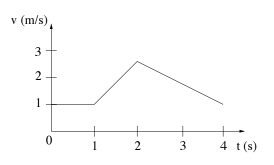🤓 Based on our data, we think this question is relevant for Professor Kezerashvili's class at BMCC CUNY.

# Solution: In the graph shown below, velocity is plotted as a function of time for an object traveling in a straight line. A) The object moves backward from 2 to 4 seconds. B) The object is at rest from 0 to 1 seconds. C) From 1 to 2 seconds the acceleration is largest. D) The object returns to where it started after 4 seconds.

###### Problem

In the graph shown below, velocity is plotted as a function of time for an object traveling in a straight line.

A) The object moves backward from 2 to 4 seconds.

B) The object is at rest from 0 to 1 seconds.

C) From 1 to 2 seconds the acceleration is largest.

D) The object returns to where it started after 4 seconds.